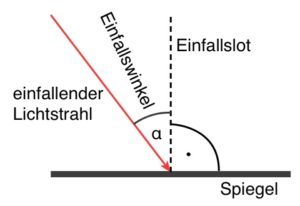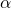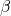# What is an angle of reflection

## Reflection on the mirror

### Reflection of light

When light hits a body or a surface, it is at least partially reflected back. This process is called reflection.

With rough surfaces, the light is reflected in all possible directions - one speaks of scattering. You already know this type of reflection from the section on the propagation of light.

Let us now investigate how light shines on a smooth surface like a mirror is reflected.

### Reflection of light on the flat mirror

A flat mirror is a smooth surface that is not curved, but completely straight ("flat"), on which most of the incident light is reflected. If a light beam hits the surface of a plane mirror, it is reflected in a certain direction.The the angle of the incident light beam, the so-called Angle of incidence("Alpha"), is placed between the incident light beam and the so-called Slot of incidence measured. The incidence plumb bob is a thin (imaginary) Auxiliary linewhich passes through the point of incidence of the light and is perpendicular to the surface. The perpendicular serves us to measure the angle of incidence:

To understand the relationship between the angle of incidence and the Reflection angle("Beta", angle of the reflected light beam), the following experiment is carried out:

#### attempt

A narrow bundle of light (“light beam”) hits a plane mirror at different angles of incidence. With the help of an angle disc, the Angle of incidenceas well as the Reflection angleto be measured:This is also used to measure the angle of reflection Slot of incidence (see above): The Reflection angleis the angle between the normal of incidence and the reflected light beam. As you can see, the incident and reflected light beam as well as the incidence perpendicular (solid line) lie in one plane.

The angle disk is now rotated with the mirror in such a way that the angles of incidence 10 °, 20 °, 30 ° ... 80 ° result one after the other. The associated reflection angles are read off and entered in a table.

#### table

 Angle of incidence0° 10° 20° 30° 40° 50° 60° 70° 80° Reflection angle0° 10° 20° 30° 40° 50° 60° 70° 80°

#### evaluation

As you can see is the angle of reflectionalways as large as the angle of incidence.

In the picture above it cannot be seen which is the incident and which is the reflected light beam. If a second light beam were to strike the mirror congruently with the reflected beam, this would be reflected congruently with the first incident light beam.

They say: The light path is reversible.

In the Reflection on the flat mirror So the following law applies: